﻿ Research on Short-term Prediction of Temperature Based on “Compact” Wavelet Neural NetworkPublications are Open
Access in this journal
Article Versions
Export Article
• Normal Style
• MLA Style
• APA Style
• Chicago Style
Research Article
Open Access Peer-reviewed

### Research on Short-term Prediction of Temperature Based on “Compact” Wavelet Neural Network

Guanghui Wang
Journal of Computer Sciences and Applications. 2020, 8(1), 5-14. DOI: 10.12691/jcsa-8-1-2
Received February 14, 2020; Revised March 18, 2020; Accepted March 27, 2020

### Abstract

The sequence prediction theory of wavelet neural network is applied to short-term temperature prediction. By using wavelet function as the activation function of the hidden layer of BP neural network, a compact wavelet neural network prediction model is established. The structural characteristics of the model are analyzed and the specific steps of building the model are described. Based on two sets of temperature observation data, internal characteristics and constraints of different data series are revealed using statistical analysis. Then, short-term temperature changes are predicted using wavelet neural network and predicted results are compared with the actual temperature. Finally, the prediction accuracy of wavelet neural network based on different data sequences is compared and analyzed. The results show that the wavelet neural network has a good accuracy for the prediction of short-term temperature change.

### 1. Introduction

In statistical sense, time series prediction belongs to conditional expectation 1, which involves solving the joint probability of current samples and past samples. However, due to the nonlinearity of conditional expectation, it is very difficult to solve the problem and the prediction model is not optimal 2. For example, the traditional AR prediction model, ARMA prediction model, and Markov chain, they are based on the stationary hypothesis of time series and cannot achieve the ideal prediction effect for non-stationary signals 3. The solution is to use the nonparametric idea of the neural network to perform prediction. Because the neural network is trained based on non-statistical hypothesis, it provides a way to solve complex problems 4. A lot of study shows that the neural network can solve the nonlinear prediction problem by loading training samples for a short time 5, 6, 7. However, the neural network has many problems such as learning efficiency, training accuracy, the selection of hidden layer nodes, and the setting of initial weights, which leads to large prediction errors. The feed-forward supervised learning network that combines the good localization property of the wavelet function with the self-learning function of artificial neural network provides an effective way to solve these problems 8.

Wavelet neural network is a new type of neural network based on the BP neural network that uses the wavelet function as activation function of hidden layers of the neural network. The biggest difference between the wavelet neural network and the BP neural network is that their activation functions of hidden layers are different. Therefore, wavelet neural network is a kind of feed-forward neural network with a feedback. Wavelet transform can make the network have fast learning, avoid data falling into the local minimum problem, and make it have wide applications. The wavelet analysis is a local analysis of time and space. It can subdivide signals into high frequency and low frequency by stretching translation. The wavelet analysis can approach any function by shifting and stretching transformation, so it has good accuracy and fault tolerance.

At present, wavelet functions and neural network can combine with each other in two ways: the first way is the "loose" combination mode in which the data signal is first processed by a wavelet analysis and the processed signal is input into neural network; the second way is the "compact" combination mode in which a wavelet basis function is used to replace many activation functions in hidden layers of neural network, this has the advantages of both the neural network and the wavelet transform. The general "compact" wavelet neural network has strong ability to process data 9.

A climate system is a highly complex nonlinear system that is coupled by many subsystems. Its changes are affected by many factors. The neural network is a nonlinear dynamic system. It is also a multi-input, multi-output or single output system. Neural network has the characteristics of nonlinear processing, self-organization and adaptability, good learning ability and association ability, etc. that is similar to the climate system 10. Since the neural network enables to deal with complex problems, it does not need to identify the physical characteristics of climate in details, does not need extensive data, and has strong fault tolerance. For incomplete, vague, and uncertain data, we can make a reasonable judgment and decision based on continuous learning and effective prediction or estimation. In this paper, the "compact" wavelet neural network system tool is used to predict the short-term temperature change. An analysis and a discussion are carried out.

### 2. Time-frequency Localization Principle of Wavelet Decomposition

2.1. The Theory of Wavelet Analysis

Wavelet analysis was developed for the deficiency of Fourier transform. Fourier transform is the most widely used analysis method in the field of signal processing. However, it has a serious disadvantage: the transform discards the practical information and the transformation result cannot judge the time when a signal occurs. This means the Fourier transform has no resolution ability in the time domain. Wavelet is a kind of waveform with limited length and an average value of 0. A wavelet function is obtained by translation and increase or decrease of the size of a parent wavelet function. Wavelet analysis is to decompose the signal into a series of wavelet functions.

The essence of using wavelet to realize multi-level decomposition of signal is to compare the original signal with a wavelet function with time-frequency domain localization function on each certain scale so as to extract wavelet coefficients in this region. In other words, a time-frequency signal is represented as a series of simple standard signals with different frequencies and delays. The core of wavelet analysis is multi-resolution, which has good localization property in time domain and frequency domain at the same time, so it can focus on any details of the signal to reveal the detailed structure of different levels of nonlinear signal. For a function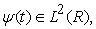if its Fourier transform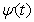satisfies the condition: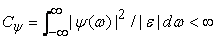(1)

thenis called the admissible wavelet generating function and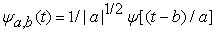is called the wavelet basis function. In practice, continuous wavelet must be discretized. The discrete wavelet function is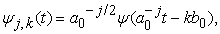and the discrete wavelet transform coefficient is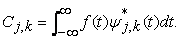(2)

The reconstruction formula for numerical calculation is 11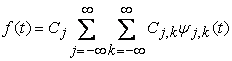(3)
2.2. Multi-resolution Analysis

The subspace of multi-resolution analysis can be approximated by a finite subspace 11: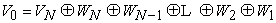(4)

The signal f (x) decomposition process is a gradual decomposition process from j to j-1 scale. The method of multi-resolution analysis is used to decompose and reconstruct the wavelet into low-frequency component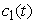and high-frequency component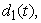and then the low-frequency componentis further decomposed so that the high-frequency component and low-frequency component on any scale can be obtained by repetition.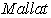tower algorithm is adopted with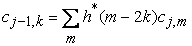(5)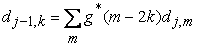(6)

The reconstruction form of wavelet coefficients can be expressed as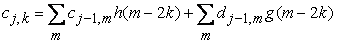(7)

### 3. Compact Wavelet Neural Network

3.1. The Structure

The wavelet generating function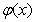that satisfies the frame condition is selected and the corresponding base function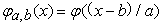is used as the activation function of the hidden layer of the neural network. The linear superposition of wavelet basis functions is used to for the representation of signals. The structure of the wavelet neural network is shown in Figure 1.

• Figure 1. Topological structure of wavelet neural network

In Figure 1,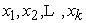are the input variables of the neural network,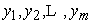are output values,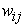and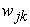are weights connecting the input layer, the hidden layer, and the output layer, respectively.is wavelet generating function. The corresponding base functionis used as the activation function of the hidden layer of the neural network. The output value of the hidden layer is: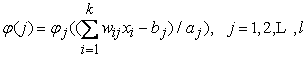(8)

In the above formulas,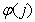is the output value of the jth node in the hidden layer;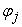is called the wavelet basis function.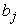is its translation factor;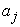is its expansion factor. In the output layer, the calculation formula of the output value is: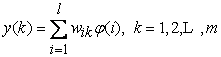(9)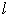is the number of nodes in the hidden layer;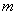is the number of nodes in the output layer.

3.2. Network Learning Method

Being similar to the weight correction algorithm of the BP neural network, the gradient correction algorithm is used to modify the weight value and wavelet base parameters of wavelet neural network, which makes the predicted output value of wavelet neural network approach the expected output value continuously. The correction process is as follows:

(1) Calculation of network prediction error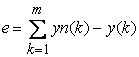where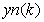is the expected output value and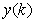is the predicted output value of wavelet neural network.

(2) Network weight correction

According to the value of prediction error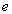, the weight of wavelet neural network and the parameters of wavelet basis function are modified by the gradient correction method. The method of increasing momentum term is used to improve the learning efficiency of the network and avoid slow evolution and falling into the minimum. In order to speed up the convergence of the network, the momentum factor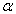of the network parameter is introduced. The iterative formula of the weight vector (or matrix) is 12: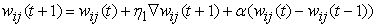(10)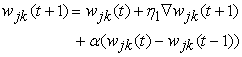(11)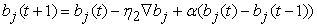(12)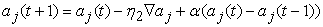(13)

Where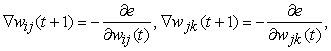(14)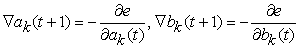(15)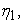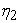is the learning efficiency,is the momentum factor,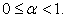When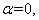it is the traditional wavelet neural network.

3.3. Training and Forecasting Methods

Add window to the time series (the length of window is the seasonal length of the series), move the window step by step (move one data position at a time), train and predict the data in the window in turn with the set wavelet neural network: according to the determined seasonal length, take the data from the first data in the sequence in turn K data as training samples and K+1 data as training targets; then move the time window backward one bit, select training samples and training targets from the second data of the sequence in the same way until enough training samples and targets are selected. The empirical formula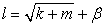(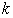is the number of input layer nodes,is the number of output layer nodes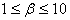) is used to determine the number of hidden layer nodes.

In summary, the specific steps of setting, training, and prediction of the wavelet neural network are as follows:

Step 1: Network initialization. Randomly initialize the scaling factor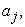translation factor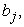network connection weight value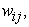andof wavelet function; set the network learning rate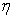and momentum factor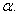Step 2: Classify the sample. The sample is divided into two parts: training sample and test sample.

The training sample is used for network training and test sample is used for testing network prediction accuracy.

Step 3: Output the predicted value. Input the training samples into the neural network, get the predicted output value of the network, and calculate the errorbetween the output value of the network and the expected output value.

Step 4: Modify the weight and parameters. According to the error, the parameters of network weight and wavelet basis function are modified to make the prediction value of network close to the expected value.

Step 5: Decide whether computation should be terminated according to the accuracy requirement.

### 4. Short Term Prediction of Temperature

Historical data show that the temperature change in an area is related to the rainfall in previous months. The design of wavelet neural network is divided into three layers: input layer, hidden layer, and output layer. Among them, the input layer inputs temperature data before the current time point, the hidden layer node is composed of the wavelet function, and the output layer outputs the predicted temperature of the current time point.

4.1. Temperature Time Series

Location of observation point: 124.48 ° E, 48.48 ° N, with observation interval of 1 hour. The observation temperature value is used as time series data, divided into two groups: group I, August 2016 (see Figure 2). Group II, January 2017 (see Figure 3). The number of data in both groups is 726. The first 654 data are used as training samples, and the left 72 data (temperature data in 3 days) are used as test samples. First, normalize the data to the correlation value between [0,1], and then carry out reversing-normalization after simulation.

4.2. Data Analysis
4.2.1. Temperature Data Distribution

Figure 2 and Figure 3 show the temperature distribution of group I data (one month in summer) and group II data (one month in winter), respectively. The data are divided into training samples and test samples. The red broken line part is the test sample set. It can be seen from Figure 2 and Figure 3 that temperature data fluctuate greatly with time, especially the data of group II.

4.2.2. Stationary Processing of Time Series

Figure 2 and Figure 3 represent the temperature distributions in summer and winter (two representative seasons of a year), respectively. Because of the non-stationarity of the sequence, it is usually used as first-order or multi-order difference to make the sequence stable before simulation and prediction. Figure 4 and Figure 5 show the difference sequence diagrams of the data in the first group and the second group, respectively. The two difference-series fluctuate around 0 with time. This means stability and the range of fluctuation is almost the same. However, it is worth noting that there is significant difference in the data difference (i.e. increment) of the test sample data range. The increment range of the test data in group I is (- 3, 1.8) while the increment range of the test data in group II is (-5, 5).

• Figure 2. Temperature data distribution of group I
• Figure 3. Temperature data distribution of group II
• Figure 4. First order difference of group I data
• Figure 5. First order difference of group II data

4.2.3. Correlation Analysis of Data Series

The calculation result shows that the correlation coefficient of the first group and the second group of data is 0.196, which indicates there is very weak correlation between the two groups of data. It means there is no similarity between the two groups of data with obvious seasonal differences. Each group of data itself can be analyzed to obtain the correlation degree of the data through the autocorrelation coefficient of the sequence. Figure 6 and Figure 7 show the trend of autocorrelation coefficient of two sets of data with the order, respectively. In Figure 6, the autocorrelation coefficient fluctuates between 0.2 and 0.5 before the order is 400, indicating that the temperature data at two different times have medium or weak correlation. In Figure 7, when the order is greater than 50, the correlation coefficient basically fluctuates between - 0.2 and 0.2, with only a few exceptions. It means the temperature data at two different times have no correlation when the order is greater than 50. This will undoubtedly affect the prediction accuracy of the model.

4.3. The Construction of Wavelet Neural Network

Because the temperature time series has the characteristics of quasi period in 24 hours, the structure of wavelet neural network is determined as 4-6-1. Four nodes in the input layer, representing the temperature value of four time points before the prediction time node; six nodes in the hidden layer; and one node in the output layer. The output value is the temperature value to be predicted. The weights of the network and the parameters of the wavelet basis function are given randomly during initialization.

Parameter selection:set the maximum iteration number of the network training as 1000, wavelet function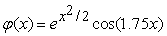(the figures of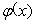and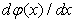are shown in Figure 8 and Figure 9, respectively), learning efficiency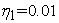and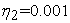, momentum factor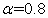, 654 training data, and 72 test data.

• Figure 6. The trend of the autocorrelation coefficient of group I
• Figure 7. The trend of the autocorrelation coefficient of group II
• Figure 8. The wavelet function
• Figure 9. The derivative of the wavelet function
4.4. Prediction Results

Wavelet neural network uses training data for 1000 iterations and the trend of prediction error in the training process is shown in Figure 10 and Figure 11. The training error decreases with the increase of the number of iterations. It means weights and parameter values are improved constantly and the prediction accuracy is improved with the increase of the number of iterations. The convergence rate of group I is faster than that of group II.

After 1000 iterations of training data, the wavelet neural network is used to predict 72 hours (3 days) of temperature change. The comparison between the predicted results and the actual temperature is shown in Figure 12 and Figure 13.

• Figure 10. Network evolution diagram of group I data
• Figure 11. Network evolution diagram of group II data

During the evolution of training data, a series of wavelet neural networks will be generated. By using the neural networks to predict the temperature change in 72 hours, many different prediction results can be obtained. The change trend of these prediction results is shown in Table 1.

With the increase of iterations of training data, the weights of wavelet neural network are constantly adjusted. It can be seen from Figure 10 and Figure 11 that the training prediction error is decreasing. It means the network output and the expected output become close with the continuous correction of the weight in the training process. Accordingly, the temperature prediction accuracy of the test data is also improved. The average error of time nodes decreases with the increase of iterations. However, it is found that when the number of iterations increases to a certain value, the error of temperature prediction does not decrease but increases. As shown in group I data in Table 1, when the number of iterations is 10000, the average absolute error of the test time node goes up to 0.6084 though the training error drops to 41.9792. There is a similar situation in group II data in Table 1. This is the outcome of over-training. It can be seen from Table 1 that the prediction accuracy of group I is significantly higher than that of group II based on the wavelet neural network method. The main reason of this difference lies in the significant difference in the fluctuation amplitude, increment change, and the autocorrelation coefficient of temperature time series (see Figure 2 - Figure 7).

• Figure 12. Comparison of prediction and actual situation (group I data)
• Figure 13. Comparison of prediction and actual situation (group II data)

### 5. Conclusion

Based on the advantages of wavelet analysis and the BP neural network, a wavelet neural network is constructed in this paper. A trained wavelet neural network is used to predict the short-term temperature and predicted results are analyzed and compared with the actual temperature. The prediction accuracy of wavelet neural networks that are trained by two groups of different temperature data is also compared. The reasons of the difference of prediction accuracy are analyzed based on the statistical characteristics of temperature data. The results show that the wavelet neural network is feasible and effective to predict the short-term temperature change. In addition, the learning efficiency of the network is improved by introducing the momentum factor in the process of modifying the weights and parameters of the wavelet neural network, which has advantages and the potential to predict short-term temperature.

### Funding

This research was supported by the National Natural Science Foundation (NSF) of China (No. 91437112).

### References

  Rizvi S A, Wang Lin-cheng, Nasrabadi N M, "Neural-network architectures for vector prediction," Proc of IEEE, 84(10)(1996) 1513-1528. In article View Article  Mellit A, Benghanem M, Kalogirou S A, "An adaptive wavlet-network model for forecasting daily total solar-radiation," Applied Energy, 83(7)(2006) 705-722. In article View Article  Guessoum A, Boubkeur S, Maafi A, "A global-irradiation model using radial basis function neural-network [C]// AAm renewable energy, energy efficiency policy and the environment," Proc of the 5th WREC. Oxford: Elsevier Sicences Lid, 1998, 332-336. In article  Kalogirou S A, "Articial neural-networks in renewable-energy systems applications, aveview," Renewable and Sustainable Energy Reviews, 54(4)(2001) 373-401. In article View Article  Hipert H S, Pedreira C E, Souza R C, “Neural-networks for short tem load forecasting: A review and evaluation,” IEEE Trans on Power Systems, 16(1)(2001) 44-55. In article View Article  Senjyu T, Takara H, Ufzata K, et al, “One-hour-ahead load forecasting using neural network,” IEEE Trans on Power Systems, 17(1)(2002) 113-118. In article View Article  Chow T W S, Leung G T, “Neural networls based short term load forecasting using weather compensation,” IEEE Trans on Power Systems, 11(4)(1996) 1736-1742. In article View Article  Zhang Qing-hua, Albert B, "Wavelet networks," IEEE Trans on Neural Networks 3(6)(1992) 889-898. In article View Article  PubMed  Luo Hang, Wand Hou-jun, Long Bing, "Research about time—-serial prediction based on tight wavelet neural-net," Application Research of Computers, 25(8)(2008) 2366-2368. In article  Guo Qingchun, Li Li, Zhang Ran, etc al, "A neural-network-based forecasting model for temperature change in China," Journal of Tropical Meteorology, 25(4) (2009) 483-487. In article  Charles K. Chui, "An introduction to wavelets," Posts & Telecom Press, 2009, pp. 13-16. In article  Shi Yan, Han Liqun, Lian Xiaoqin, "Neural network design method and case analysis," Beijing University of Posts and Telecommunications Press，2009, pp. 25-28. In articleThis work is licensed under a Creative Commons Attribution 4.0 International License. To view a copy of this license, visit http://creativecommons.org/licenses/by/4.0/